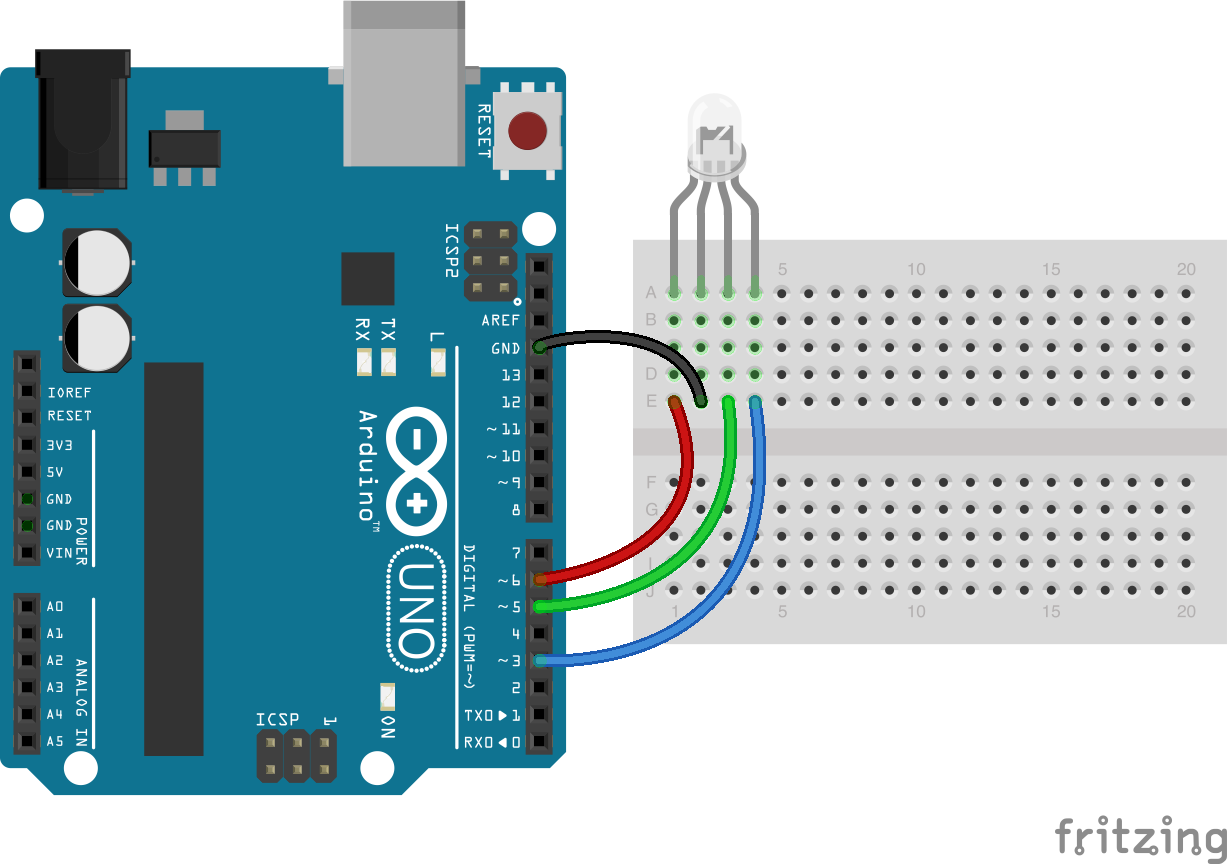Demonstrates use of an RGB LED (common cathode) by setting its color to red (`#ff0000`) and making it blink. Requires RGB LED on pins that support PWM (usually denoted by ~).

##### Common Cathode RGB LED. (Arduino UNO)

RGB LED connected to pins 6, 5, and 3 for red, green, and blue respectively. The common pin is connected to ground.Fritzing diagram: led-rgb.fzz

Run this example from the command line with:

``````node eg/led-rgb.js
``````
``````const { Board, Led } = require("johnny-five");
const board = new Board();

// Initialize the RGB LED
const led = new Led.RGB({
pins: {
red: 6,
green: 5,
blue: 3
}
});

// RGB LED alternate constructor
// This will normalize an array of pins in [r, g, b]
// order to an object (like above) that's shaped like:
// {
//   red: r,
//   green: g,
//   blue: b
// }
// const led = new Led.RGB([3,5,6]);

// Add led to REPL (optional)
board.repl.inject({ led });

// Turn it on and set the initial color
led.on();
led.color("#FF0000");